# Math help from the Learning Centre

This guide provides useful resources for a wide variety of math topics. It is targeted at students enrolled in a math course or any other Centennial course that requires math knowledge and skills.

## Introduction

Transformations are a way for us to take elementary functions and move them around throughout the cartesian plane. There are three distinct categories of transformations. Namely:

• translations, which move the function up, down, left or right
• reflections, which reflect the function over a given axis
• stretches, which stretch or compress the function by a factor of a given number.

Transformations can be applied to many aspects of real life including architecture, aviation, engineering, and even simple day-to-day tasks like looking in a mirror or turning on a faucet.

## Vertical Translations

Vertical translations are what causes a function to move up and down.

Given an elementary function $$y=f(x)$$, simply adding a number c to the function will cause it to move c units UP. This looks like $$y=f(x)+c$$.

To move a function DOWN c units, we subtract the number c from the function as follows: $$y=f(x)−c$$.

Example:

Consider the elementary function $$y=x^2$$. Look at what happens to the graph when we add or subtract 3 from the function:

The graph of $$y=x^2$$: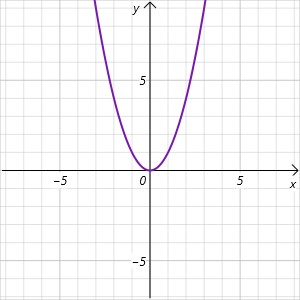The graph of $$y=x^2+3$$ moves the function 3 units up: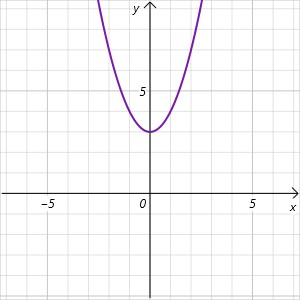The graph of $$y=x^2-3$$ moves the function 3 units down: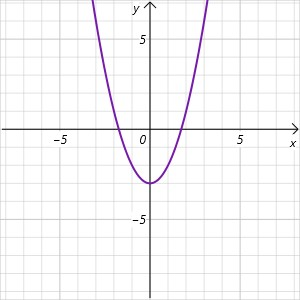## Horizontal Translations

We can translate a function horizontally (left and right) by adding or subtracting a number c to the x-coordinate inside the function.

Adding c to the x-coordinate $$y=f(x+c)$$ will result in the function moving LEFT c units.

Subtracting c from the x-coordinate $$y=f(x-c)$$ will move the functions RIGHT c units.

Example:

Consider the elementary function $$y=\sqrt{x}$$.  Look at what happens when we add or subtract 2 to the x-coordinate within the function:

The graph of $$y=\sqrt{x}$$: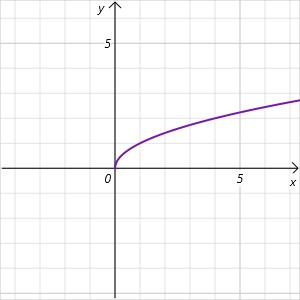The graph of $$y=\sqrt{x+2}$$ moves the function 2 units to the left: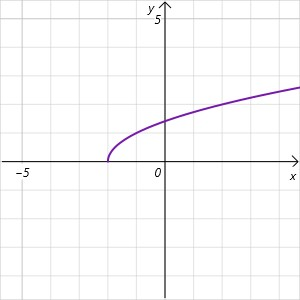The graph of $$y=\sqrt{x-2}$$ moves the function 2 units to the right: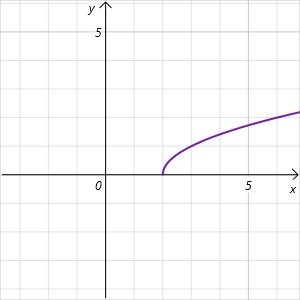## Reflections

Reflections over the x-axis are represented by negating the function as follows:

$$y=−f(x)$$

Reflections over the y-axis are represented by negating the x-coordinate within the function as follows:

$$y=f(−x)$$

Example:

Consider the elementary function $$y=2^x$$. Observe what happens when we negate the function or the x-coordinate:

The graph of $$y=2^x$$: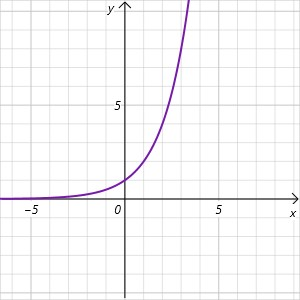The graph of $$y=-2^x$$ results in a reflection over the X-AXIS: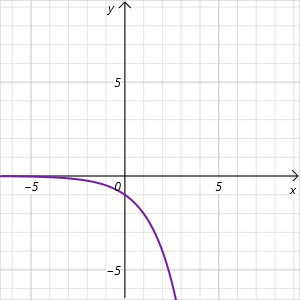The graph of $$y=2^{-x}$$ results in a reflection over the Y-AXIS: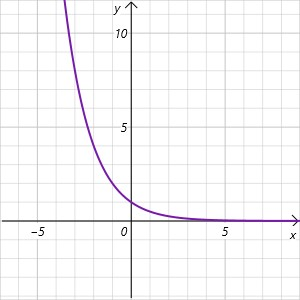## Vertical Stretches/Compressions

We express vertical stretches and compressions by multiplying the whole function by a number. If the number is greater than 1, we have a VERTICAL STRETCH but if it's less than 1, we have a VERTICAL COMPRESSION.

$$y=cf(x)$$ represents a vertical stretch by a factor of c

$$y=\frac{1}{c}f(x)$$ represents a vertical compression by a factor of c

Example:

Consider again the elementary function $$y=\sqrt{x}$$. Observe what happens when we multiply the function by $$4$$ and $$\frac{1}{4}$$

The graph of $$y=\sqrt{x}$$: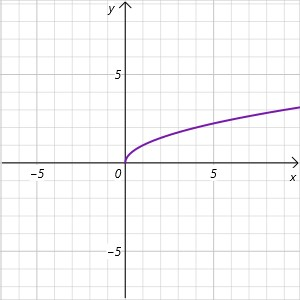The graph of $$y=4\sqrt{x}$$ vertically stretches the function by a factor of 4: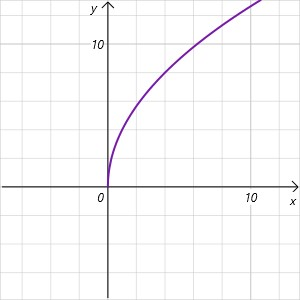The graph of $$y=\frac{1}{4}\sqrt{x}$$ vertically compresses the function by a factor of 4: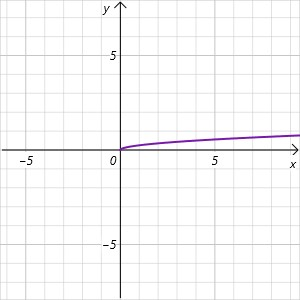## Horizontal Stretches/Compressions

For horizontal stretches and compressions, we multiply the x-coordinate within the function by a number. If the number is greater than 1, it's a HORIZONTAL COMPRESSION and if the number is less than 1, it's a HORIZONTAL STRETCH.

$$y=f(cx)$$ represents a horizontal compression by a factor of c and

$$y=f(\frac{x}{c})$$ represents a horizontal stretch by a factor of c.

Example:

Consider the elementary function $$y=x^3$$. Observe what happens to the graphs when we multiply the x-coordinate by $$3$$ and $$\frac{1}{3}$$.

The graph of $$y=x^3$$: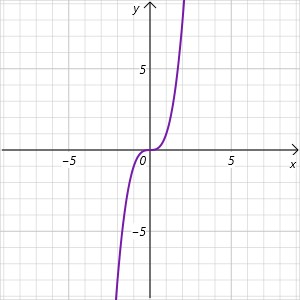The graph of $$y=(3x)^3$$ horizontally compresses the function by a factor of 3: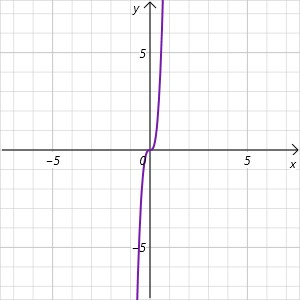The graph of $$y=(\frac{x}{3})^3$$ horizontally stretches the function by a factor of 3: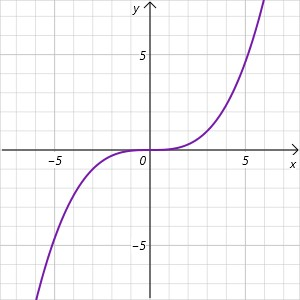## Putting it all together

When sketching an elementary function that has undergone multiple transformations, we must carry out those transformations in a certain order. We first perform stretches, then reflections, and finally translations. An easy way to remember this order is simply thinking about the order of events that happen in the morning. The first thing someone does when they wake up is STRETCH, then they brush  their teeth in a mirror with a REFLECTION, and finally they TRANSLATE themselves to either work or school.

## Summary

Transformation Description
$$y=f(x)+c$$ Moves the function c units up
$$y=f(x)-c$$ Moves the function c units down
$$y=f(x+c)$$ Moves the function c units left
$$y=f(x-c)$$ Moves the function c units right
$$y=-f(x)$$ Reflects the function over the x-axis
$$y=f(-x)$$ Reflects the function over the y-axis
$$y=cf(x)$$ Stretches the function vertically by a factor of c
$$y=\frac{1}{c}f(x)$$ Compresses the function vertically by a factor of c
$$y=f(cx)$$ Compresses the function horizontally by a factor of c
$$y=f(\frac{x}{c})$$ Stretches the function horizontally by a factor of c

## Practice

Graph the following functions. You can check your answer by graphing the function on a graphing software such as Desmos or Geogebra:

$$y=5(logx+3)−2$$

$$y=−3\sqrt{2x+1}-8$$

$$y=−5x^3+4$$